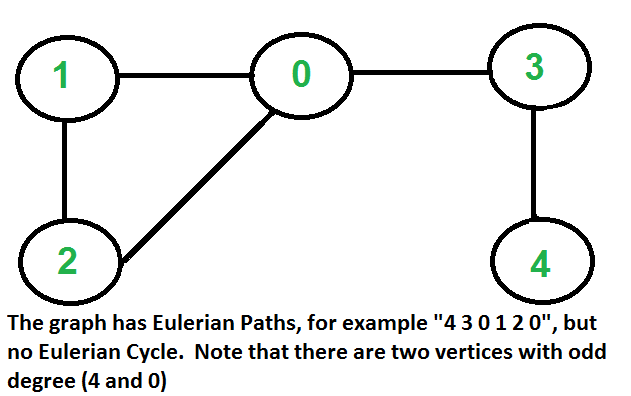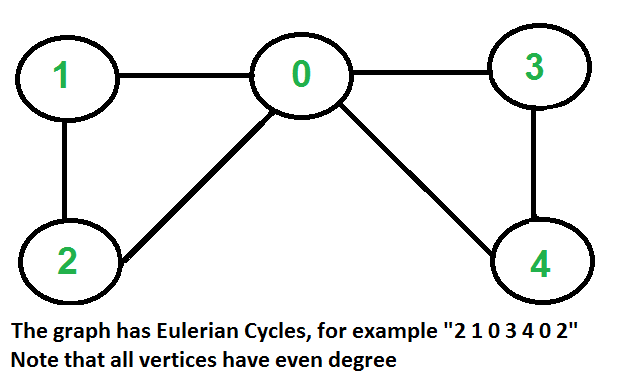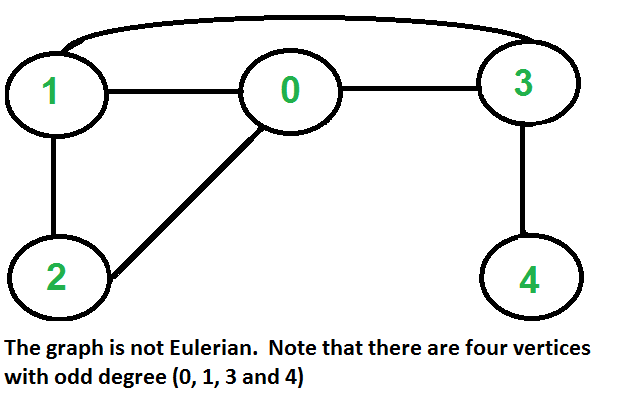GFG App
Open AppBrowser
Continue

# Eulerian path and circuit for undirected graph

Eulerian Path is a path in a graph that visits every edge exactly once. Eulerian Circuit is an Eulerian Path that starts and ends on the same vertex.How to find whether a given graph is Eulerian or not?

The problem is same as following question. “Is it possible to draw a given graph without lifting pencil from the paper and without tracing any of the edges more than once”.
A graph is called Eulerian if it has an Eulerian Cycle and called Semi-Eulerian if it has an Eulerian Path. The problem seems similar to Hamiltonian Path which is NP complete problem for a general graph. Fortunately, we can find whether a given graph has a Eulerian Path or not in polynomial time. In fact, we can find it in O(V+E) time.
Following are some interesting properties of undirected graphs with an Eulerian path and cycle. We can use these properties to find whether a graph is Eulerian or not.

Eulerian Cycle: An undirected graph has Eulerian cycle if following two conditions are true.

1. All vertices with non-zero degree are connected. We don’t care about vertices with zero degree because they don’t belong to Eulerian Cycle or Path (we only consider all edges).
2. All vertices have even degree.

Eulerian Path: An undirected graph has Eulerian Path if following two conditions are true.

1. Same as condition (a) for Eulerian Cycle.
2. If zero or two vertices have odd degree and all other vertices have even degree. Note that only one vertex with odd degree is not possible in an undirected graph (sum of all degrees is always even in an undirected graph)

Note that a graph with no edges is considered Eulerian because there are no edges to traverse.

How does this work?
In Eulerian path, each time we visit a vertex v, we walk through two unvisited edges with one end point as v. Therefore, all middle vertices in Eulerian Path must have even degree. For Eulerian Cycle, any vertex can be middle vertex, therefore all vertices must have even degree.

Recommended Practice

Implementation:

## C++

 `// A C++ program to check if a given graph is Eulerian or not` `#include` `#include ` `using` `namespace` `std;`   `// A class that represents an undirected graph` `class` `Graph` `{` `    ``int` `V;    ``// No. of vertices` `    ``list<``int``> *adj;    ``// A dynamic array of adjacency lists` `public``:` `    ``// Constructor and destructor` `    ``Graph(``int` `V)   {``this``->V = V; adj = ``new` `list<``int``>[V]; }` `    ``~Graph() { ``delete` `[] adj; } ``// To avoid memory leak`   `     ``// function to add an edge to graph` `    ``void` `addEdge(``int` `v, ``int` `w);`   `    ``// Method to check if this graph is Eulerian or not` `    ``int` `isEulerian();`   `    ``// Method to check if all non-zero degree vertices are connected` `    ``bool` `isConnected();`   `    ``// Function to do DFS starting from v. Used in isConnected();` `    ``void` `DFSUtil(``int` `v, ``bool` `visited[]);` `};`   `void` `Graph::addEdge(``int` `v, ``int` `w)` `{` `    ``adj[v].push_back(w);` `    ``adj[w].push_back(v);  ``// Note: the graph is undirected` `}`   `void` `Graph::DFSUtil(``int` `v, ``bool` `visited[])` `{` `    ``// Mark the current node as visited and print it` `    ``visited[v] = ``true``;`   `    ``// Recur for all the vertices adjacent to this vertex` `    ``list<``int``>::iterator i;` `    ``for` `(i = adj[v].begin(); i != adj[v].end(); ++i)` `        ``if` `(!visited[*i])` `            ``DFSUtil(*i, visited);` `}`   `// Method to check if all non-zero degree vertices are connected.` `// It mainly does DFS traversal starting from` `bool` `Graph::isConnected()` `{` `    ``// Mark all the vertices as not visited` `    ``bool` `visited[V];` `    ``int` `i;` `    ``for` `(i = 0; i < V; i++)` `        ``visited[i] = ``false``;`   `    ``// Find a vertex with non-zero degree` `    ``for` `(i = 0; i < V; i++)` `        ``if` `(adj[i].size() != 0)` `            ``break``;`   `    ``// If there are no edges in the graph, return true` `    ``if` `(i == V)` `        ``return` `true``;`   `    ``// Start DFS traversal from a vertex with non-zero degree` `    ``DFSUtil(i, visited);`   `    ``// Check if all non-zero degree vertices are visited` `    ``for` `(i = 0; i < V; i++)` `       ``if` `(visited[i] == ``false` `&& adj[i].size() > 0)` `            ``return` `false``;`   `    ``return` `true``;` `}`   `/* The function returns one of the following values` `   ``0 --> If graph is not Eulerian` `   ``1 --> If graph has an Euler path (Semi-Eulerian)` `   ``2 --> If graph has an Euler Circuit (Eulerian)  */` `int` `Graph::isEulerian()` `{` `    ``// Check if all non-zero degree vertices are connected` `    ``if` `(isConnected() == ``false``)` `        ``return` `0;`   `    ``// Count vertices with odd degree` `    ``int` `odd = 0;` `    ``for` `(``int` `i = 0; i < V; i++)` `        ``if` `(adj[i].size() & 1)` `            ``odd++;`   `    ``// If count is more than 2, then graph is not Eulerian` `    ``if` `(odd > 2)` `        ``return` `0;`   `    ``// If odd count is 2, then semi-eulerian.` `    ``// If odd count is 0, then eulerian` `    ``// Note that odd count can never be 1 for undirected graph` `    ``return` `(odd)? 1 : 2;` `}`   `// Function to run test cases` `void` `test(Graph &g)` `{` `    ``int` `res = g.isEulerian();` `    ``if` `(res == 0)` `        ``cout << ``"graph is not Eulerian\n"``;` `    ``else` `if` `(res == 1)` `        ``cout << ``"graph has a Euler path\n"``;` `    ``else` `        ``cout << ``"graph has a Euler cycle\n"``;` `}`   `// Driver program to test above function` `int` `main()` `{` `    ``// Let us create and test graphs shown in above figures` `    ``Graph g1(5);` `    ``g1.addEdge(1, 0);` `    ``g1.addEdge(0, 2);` `    ``g1.addEdge(2, 1);` `    ``g1.addEdge(0, 3);` `    ``g1.addEdge(3, 4);` `    ``test(g1);`   `    ``Graph g2(5);` `    ``g2.addEdge(1, 0);` `    ``g2.addEdge(0, 2);` `    ``g2.addEdge(2, 1);` `    ``g2.addEdge(0, 3);` `    ``g2.addEdge(3, 4);` `    ``g2.addEdge(4, 0);` `    ``test(g2);`   `    ``Graph g3(5);` `    ``g3.addEdge(1, 0);` `    ``g3.addEdge(0, 2);` `    ``g3.addEdge(2, 1);` `    ``g3.addEdge(0, 3);` `    ``g3.addEdge(3, 4);` `    ``g3.addEdge(1, 3);` `    ``test(g3);`   `    ``// Let us create a graph with 3 vertices` `    ``// connected in the form of cycle` `    ``Graph g4(3);` `    ``g4.addEdge(0, 1);` `    ``g4.addEdge(1, 2);` `    ``g4.addEdge(2, 0);` `    ``test(g4);`   `    ``// Let us create a graph with all vertices` `    ``// with zero degree` `    ``Graph g5(3);` `    ``test(g5);`   `    ``return` `0;` `}`

## Java

 `// A Java program to check if a given graph is Eulerian or not` `import` `java.io.*;` `import` `java.util.*;` `import` `java.util.LinkedList;`   `// This class represents an undirected graph using adjacency list` `// representation` `class` `Graph` `{` `    ``private` `int` `V;   ``// No. of vertices`   `    ``// Array  of lists for Adjacency List Representation` `    ``private` `LinkedList adj[];`   `    ``// Constructor` `    ``Graph(``int` `v)` `    ``{` `        ``V = v;` `        ``adj = ``new` `LinkedList[v];` `        ``for` `(``int` `i=``0``; i i = adj[v].listIterator();` `        ``while` `(i.hasNext())` `        ``{` `            ``int` `n = i.next();` `            ``if` `(!visited[n])` `                ``DFSUtil(n, visited);` `        ``}` `    ``}`   `    ``// Method to check if all non-zero degree vertices are` `    ``// connected. It mainly does DFS traversal starting from` `    ``boolean` `isConnected()` `    ``{` `        ``// Mark all the vertices as not visited` `        ``boolean` `visited[] = ``new` `boolean``[V];` `        ``int` `i;` `        ``for` `(i = ``0``; i < V; i++)` `            ``visited[i] = ``false``;`   `        ``// Find a vertex with non-zero degree` `        ``for` `(i = ``0``; i < V; i++)` `            ``if` `(adj[i].size() != ``0``)` `                ``break``;`   `        ``// If there are no edges in the graph, return true` `        ``if` `(i == V)` `            ``return` `true``;`   `        ``// Start DFS traversal from a vertex with non-zero degree` `        ``DFSUtil(i, visited);`   `        ``// Check if all non-zero degree vertices are visited` `        ``for` `(i = ``0``; i < V; i++)` `           ``if` `(visited[i] == ``false` `&& adj[i].size() > ``0``)` `                ``return` `false``;`   `        ``return` `true``;` `    ``}`   `    ``/* The function returns one of the following values` `       ``0 --> If graph is not Eulerian` `       ``1 --> If graph has an Euler path (Semi-Eulerian)` `       ``2 --> If graph has an Euler Circuit (Eulerian)  */` `    ``int` `isEulerian()` `    ``{` `        ``// Check if all non-zero degree vertices are connected` `        ``if` `(isConnected() == ``false``)` `            ``return` `0``;`   `        ``// Count vertices with odd degree` `        ``int` `odd = ``0``;` `        ``for` `(``int` `i = ``0``; i < V; i++)` `            ``if` `(adj[i].size()%``2``!=``0``)` `                ``odd++;`   `        ``// If count is more than 2, then graph is not Eulerian` `        ``if` `(odd > ``2``)` `            ``return` `0``;`   `        ``// If odd count is 2, then semi-eulerian.` `        ``// If odd count is 0, then eulerian` `        ``// Note that odd count can never be 1 for undirected graph` `        ``return` `(odd==``2``)? ``1` `: ``2``;` `    ``}`   `    ``// Function to run test cases` `    ``void` `test()` `    ``{` `        ``int` `res = isEulerian();` `        ``if` `(res == ``0``)` `            ``System.out.println(``"graph is not Eulerian"``);` `        ``else` `if` `(res == ``1``)` `            ``System.out.println(``"graph has a Euler path"``);` `        ``else` `           ``System.out.println(``"graph has a Euler cycle"``);` `    ``}`   `    ``// Driver method` `    ``public` `static` `void` `main(String args[])` `    ``{` `        ``// Let us create and test graphs shown in above figures` `        ``Graph g1 = ``new` `Graph(``5``);` `        ``g1.addEdge(``1``, ``0``);` `        ``g1.addEdge(``0``, ``2``);` `        ``g1.addEdge(``2``, ``1``);` `        ``g1.addEdge(``0``, ``3``);` `        ``g1.addEdge(``3``, ``4``);` `        ``g1.test();`   `        ``Graph g2 = ``new` `Graph(``5``);` `        ``g2.addEdge(``1``, ``0``);` `        ``g2.addEdge(``0``, ``2``);` `        ``g2.addEdge(``2``, ``1``);` `        ``g2.addEdge(``0``, ``3``);` `        ``g2.addEdge(``3``, ``4``);` `        ``g2.addEdge(``4``, ``0``);` `        ``g2.test();`   `        ``Graph g3 = ``new` `Graph(``5``);` `        ``g3.addEdge(``1``, ``0``);` `        ``g3.addEdge(``0``, ``2``);` `        ``g3.addEdge(``2``, ``1``);` `        ``g3.addEdge(``0``, ``3``);` `        ``g3.addEdge(``3``, ``4``);` `        ``g3.addEdge(``1``, ``3``);` `        ``g3.test();`   `        ``// Let us create a graph with 3 vertices` `        ``// connected in the form of cycle` `        ``Graph g4 = ``new` `Graph(``3``);` `        ``g4.addEdge(``0``, ``1``);` `        ``g4.addEdge(``1``, ``2``);` `        ``g4.addEdge(``2``, ``0``);` `        ``g4.test();`   `        ``// Let us create a graph with all vertices` `        ``// with zero degree` `        ``Graph g5 = ``new` `Graph(``3``);` `        ``g5.test();` `    ``}` `}` `// This code is contributed by Aakash Hasija`

## Python3

 `# Python program to check if a given graph is Eulerian or not` `#Complexity : O(V+E)`   `from` `collections ``import` `defaultdict`   `# This class represents a undirected graph using adjacency list representation`     `class` `Graph:`   `    ``def` `__init__(``self``, vertices):` `        ``self``.V ``=` `vertices  ``# No. of vertices` `        ``self``.graph ``=` `defaultdict(``list``)  ``# default dictionary to store graph`   `    ``# function to add an edge to graph` `    ``def` `addEdge(``self``, u, v):` `        ``self``.graph[u].append(v)` `        ``self``.graph[v].append(u)`   `    ``# A function used by isConnected` `    ``def` `DFSUtil(``self``, v, visited):` `        ``# Mark the current node as visited` `        ``visited[v] ``=` `True`   `        ``# Recur for all the vertices adjacent to this vertex` `        ``for` `i ``in` `self``.graph[v]:` `            ``if` `visited[i] ``=``=` `False``:` `                ``self``.DFSUtil(i, visited)`   `    ``'''Method to check if all non-zero degree vertices are` `    ``connected. It mainly does DFS traversal starting from ` `    ``node with non-zero degree'''`   `    ``def` `isConnected(``self``):`   `        ``# Mark all the vertices as not visited` `        ``visited ``=` `[``False``]``*``(``self``.V)`   `        ``#  Find a vertex with non-zero degree` `        ``for` `i ``in` `range``(``self``.V):` `            ``if` `len``(``self``.graph[i]) !``=` `0``:` `                ``break`   `        ``# If there are no edges in the graph, return true` `        ``if` `i ``=``=` `self``.V``-``1``:` `            ``return` `True`   `        ``# Start DFS traversal from a vertex with non-zero degree` `        ``self``.DFSUtil(i, visited)`   `        ``# Check if all non-zero degree vertices are visited` `        ``for` `i ``in` `range``(``self``.V):` `            ``if` `visited[i] ``=``=` `False` `and` `len``(``self``.graph[i]) > ``0``:` `                ``return` `False`   `        ``return` `True`   `    ``'''The function returns one of the following values` `       ``0 --> If graph is not Eulerian` `       ``1 --> If graph has an Euler path (Semi-Eulerian)` `       ``2 --> If graph has an Euler Circuit (Eulerian)  '''`   `    ``def` `isEulerian(``self``):` `        ``# Check if all non-zero degree vertices are connected` `        ``if` `self``.isConnected() ``=``=` `False``:` `            ``return` `0` `        ``else``:` `            ``# Count vertices with odd degree` `            ``odd ``=` `0` `            ``for` `i ``in` `range``(``self``.V):` `                ``if` `len``(``self``.graph[i]) ``%` `2` `!``=` `0``:` `                    ``odd ``+``=` `1`   `            ``'''If odd count is 2, then semi-eulerian.` `            ``If odd count is 0, then eulerian` `            ``If count is more than 2, then graph is not Eulerian` `            ``Note that odd count can never be 1 for undirected graph'''` `            ``if` `odd ``=``=` `0``:` `                ``return` `2` `            ``elif` `odd ``=``=` `2``:` `                ``return` `1` `            ``elif` `odd > ``2``:` `                ``return` `0`   `     ``# Function to run test cases`   `    ``def` `test(``self``):` `        ``res ``=` `self``.isEulerian()` `        ``if` `res ``=``=` `0``:` `            ``print``(``"graph is not Eulerian"``)` `        ``elif` `res ``=``=` `1``:` `            ``print``(``"graph has a Euler path"``)` `        ``else``:` `            ``print``(``"graph has a Euler cycle"``)`     `# Let us create and test graphs shown in above figures` `g1 ``=` `Graph(``5``)` `g1.addEdge(``1``, ``0``)` `g1.addEdge(``0``, ``2``)` `g1.addEdge(``2``, ``1``)` `g1.addEdge(``0``, ``3``)` `g1.addEdge(``3``, ``4``)` `g1.test()`   `g2 ``=` `Graph(``5``)` `g2.addEdge(``1``, ``0``)` `g2.addEdge(``0``, ``2``)` `g2.addEdge(``2``, ``1``)` `g2.addEdge(``0``, ``3``)` `g2.addEdge(``3``, ``4``)` `g2.addEdge(``4``, ``0``)` `g2.test()`   `g3 ``=` `Graph(``5``)` `g3.addEdge(``1``, ``0``)` `g3.addEdge(``0``, ``2``)` `g3.addEdge(``2``, ``1``)` `g3.addEdge(``0``, ``3``)` `g3.addEdge(``3``, ``4``)` `g3.addEdge(``1``, ``3``)` `g3.test()`   `# Let us create a graph with 3 vertices` `# connected in the form of cycle` `g4 ``=` `Graph(``3``)` `g4.addEdge(``0``, ``1``)` `g4.addEdge(``1``, ``2``)` `g4.addEdge(``2``, ``0``)` `g4.test()`   `# Let us create a graph with all vertices` `# with zero degree` `g5 ``=` `Graph(``3``)` `g5.test()`   `# This code is contributed by Neelam Yadav`

## C#

 `// A C# program to check if a given graph is Eulerian or not` `using` `System;` `using` `System.Collections.Generic;`   ` `  `// This class represents an undirected graph using adjacency list` `// representation` `public` `class` `Graph` `{` `    ``private` `int` `V;   ``// No. of vertices` ` `  `    ``// Array  of lists for Adjacency List Representation` `    ``private` `List<``int``> []adj;` ` `  `    ``// Constructor` `    ``Graph(``int` `v)` `    ``{` `        ``V = v;` `        ``adj = ``new` `List<``int``>[v];` `        ``for` `(``int` `i=0; i();` `    ``}` ` `  `    ``//Function to add an edge into the graph` `    ``void` `addEdge(``int` `v, ``int` `w)` `    ``{` `        ``adj[v].Add(w);``// Add w to v's list.` `        ``adj[w].Add(v); ``//The graph is undirected` `    ``}` ` `  `    ``// A function used by DFS` `    ``void` `DFSUtil(``int` `v,``bool` `[]visited)` `    ``{` `        ``// Mark the current node as visited` `        ``visited[v] = ``true``;` ` `  `        ``// Recur for all the vertices adjacent to this vertex` `        ``foreach``(``int` `i ``in` `adj[v]){` `            ``int` `n = i;` `            ``if` `(!visited[n])` `                ``DFSUtil(n, visited);` `        ``}` `    ``}` ` `  `    ``// Method to check if all non-zero degree vertices are` `    ``// connected. It mainly does DFS traversal starting from` `    ``bool` `isConnected()` `    ``{` `        ``// Mark all the vertices as not visited` `        ``bool` `[]visited = ``new` `bool``[V];` `        ``int` `i;` `        ``for` `(i = 0; i < V; i++)` `            ``visited[i] = ``false``;` ` `  `        ``// Find a vertex with non-zero degree` `        ``for` `(i = 0; i < V; i++)` `            ``if` `(adj[i].Count != 0)` `                ``break``;` ` `  `        ``// If there are no edges in the graph, return true` `        ``if` `(i == V)` `            ``return` `true``;` ` `  `        ``// Start DFS traversal from a vertex with non-zero degree` `        ``DFSUtil(i, visited);` ` `  `        ``// Check if all non-zero degree vertices are visited` `        ``for` `(i = 0; i < V; i++)` `           ``if` `(visited[i] == ``false` `&& adj[i].Count > 0)` `                ``return` `false``;` ` `  `        ``return` `true``;` `    ``}` ` `  `    ``/* The function returns one of the following values` `       ``0 --> If graph is not Eulerian` `       ``1 --> If graph has an Euler path (Semi-Eulerian)` `       ``2 --> If graph has an Euler Circuit (Eulerian)  */` `    ``int` `isEulerian()` `    ``{` `        ``// Check if all non-zero degree vertices are connected` `        ``if` `(isConnected() == ``false``)` `            ``return` `0;` ` `  `        ``// Count vertices with odd degree` `        ``int` `odd = 0;` `        ``for` `(``int` `i = 0; i < V; i++)` `            ``if` `(adj[i].Count%2!=0)` `                ``odd++;` ` `  `        ``// If count is more than 2, then graph is not Eulerian` `        ``if` `(odd > 2)` `            ``return` `0;` ` `  `        ``// If odd count is 2, then semi-eulerian.` `        ``// If odd count is 0, then eulerian` `        ``// Note that odd count can never be 1 for undirected graph` `        ``return` `(odd==2)? 1 : 2;` `    ``}` ` `  `    ``// Function to run test cases` `    ``void` `test()` `    ``{` `        ``int` `res = isEulerian();` `        ``if` `(res == 0)` `            ``Console.WriteLine(``"graph is not Eulerian"``);` `        ``else` `if` `(res == 1)` `            ``Console.WriteLine(``"graph has a Euler path"``);` `        ``else` `           ``Console.WriteLine(``"graph has a Euler cycle"``);` `    ``}` ` `  `    ``// Driver method` `    ``public` `static` `void` `Main(String []args)` `    ``{` `        ``// Let us create and test graphs shown in above figures` `        ``Graph g1 = ``new` `Graph(5);` `        ``g1.addEdge(1, 0);` `        ``g1.addEdge(0, 2);` `        ``g1.addEdge(2, 1);` `        ``g1.addEdge(0, 3);` `        ``g1.addEdge(3, 4);` `        ``g1.test();` ` `  `        ``Graph g2 = ``new` `Graph(5);` `        ``g2.addEdge(1, 0);` `        ``g2.addEdge(0, 2);` `        ``g2.addEdge(2, 1);` `        ``g2.addEdge(0, 3);` `        ``g2.addEdge(3, 4);` `        ``g2.addEdge(4, 0);` `        ``g2.test();` ` `  `        ``Graph g3 = ``new` `Graph(5);` `        ``g3.addEdge(1, 0);` `        ``g3.addEdge(0, 2);` `        ``g3.addEdge(2, 1);` `        ``g3.addEdge(0, 3);` `        ``g3.addEdge(3, 4);` `        ``g3.addEdge(1, 3);` `        ``g3.test();` ` `  `        ``// Let us create a graph with 3 vertices` `        ``// connected in the form of cycle` `        ``Graph g4 = ``new` `Graph(3);` `        ``g4.addEdge(0, 1);` `        ``g4.addEdge(1, 2);` `        ``g4.addEdge(2, 0);` `        ``g4.test();` ` `  `        ``// Let us create a graph with all vertices` `        ``// with zero degree` `        ``Graph g5 = ``new` `Graph(3);` `        ``g5.test();` `    ``}` `}`   `// This code contributed by PrinciRaj1992`

## Javascript

 ``

Output

```graph has a Euler path
graph has a Euler cycle
graph is not Eulerian
graph has a Euler cycle
graph has a Euler cycle```

Time Complexity: O(V+E)

Space Complexity: O(V+E)

My Personal Notes arrow_drop_up